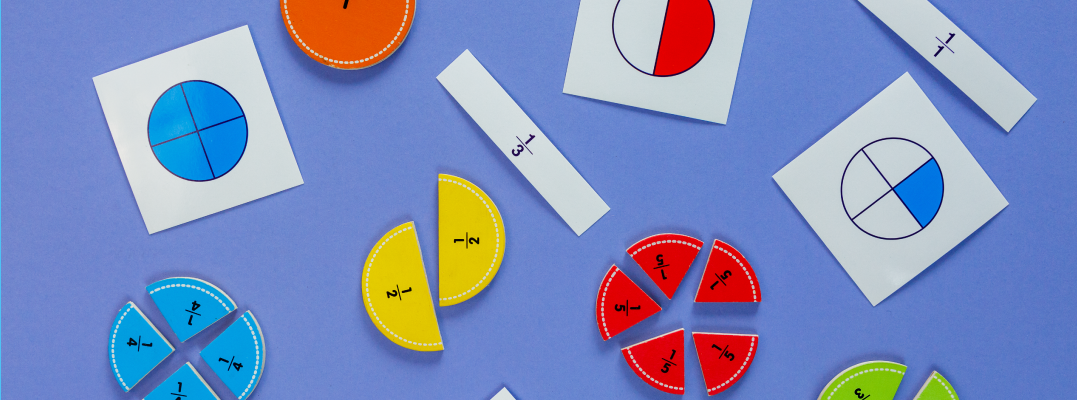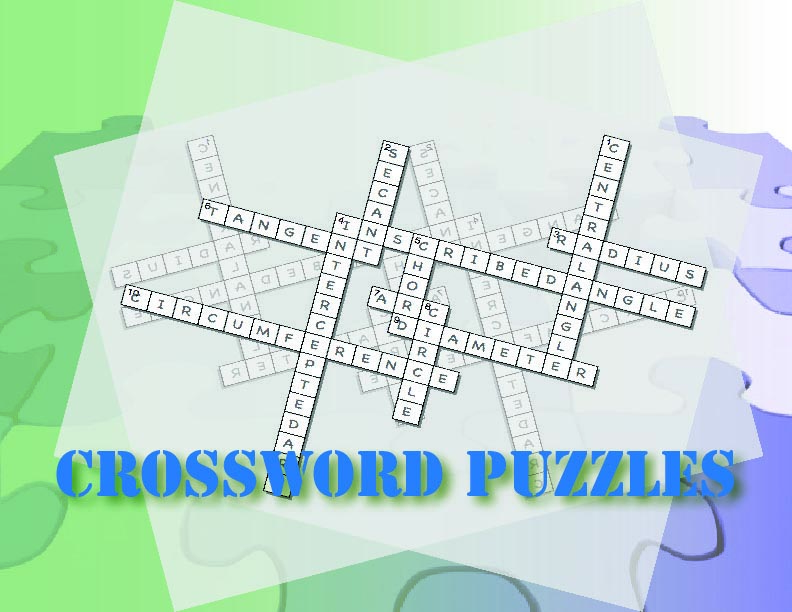# Unit: Introduction to Fractions### The suggested timeframe for each lesson can be adapted to suit your needs.### Days 1-2: Speaking Math

Introduction of key vocabulary around fractions. This is a gamified experience with word puzzles and other games.

Introduce these terms and definitions

• Fraction
• Numerator
• Denominator
• Equivalent Fraction
• Simplest form
• Proper Fraction
• Improper Fraction
• Mixed Number
• Least Common Denominator
• Unit Fraction

Media4Math Resources:

Goal: A low-stress intro to fractions vocabulary. These two days lay the groundwork of vocabulary and basic concepts. This also reinforces the notion that math is a language, an understandable one that students can comprehend.### Days 3-4: Building Blocks

Introduction to the fractions in simplest form. Students go through a tutorial on the properties of fractions in simplest form. Then they play a game called Pizza Party in which they determine the amount of pizza left over. Level 2 of this game has students determining the fraction of pizza eaten.

Media4Math Resources:

Goal: To develop a concrete understanding of fractions in simplest form.### Days 5-6: Putting it Together

Students learn about unit fractions, proper fractions, and improper fractions.

Media4Math Resources:

Goal: To expand on the definition of a fraction to include unit, proper, improper, and equivalent fractions.### Days 7-8: Show What You Know

Use the tutorial and worksheet to develop and practice the skills of identifying and generating equivalent fractions.This student module includes assessment items. You can assign this module to individual students and create a dashboard to track student progress.

Media4Math Resources:

Goal: Assess student mastery of basic fraction concepts.